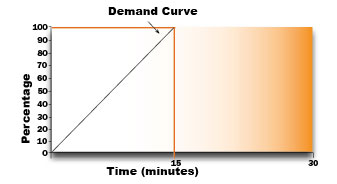## Mechanical Demand Meter

A demand meter is equipped with two pointers (one red which indicates your actual/real time demand and one black pointer which indicates your peak since the last meter reading) which register the demand or the maximum rate at which electrical energy is delivered. A thermal coil controls the red pointer, and as the energy passes through the meter, the coil heats, expands and moves the red pointer up the scale. When the red pointer advances, it pushes the black pointer forward at the same rate. However, when the red pointer retreats, the black pointer remains stationary, indicating the maximum demand. It should be noted that this heating and cooling of the coil does not happen instantaneously. There is a time lag as per the following curve. See Diagram A.

Diagram ANote: The black pointer is manually reset by the meter reader to the red pointer after the reading is recorded each month and then re-sealed by the meter reader.

## Electronic and Hybrid Meter

Rolling Block Demand

The 15 minute demand interval is broken down into three 5 minute subintervals. The meter calculates the average load in each 5 minute subinterval as well as the average of the three 5 minutes subinterval to calculate the 15 minute billing demand. It’s called the rolling block because the demand is updated every 5 minutes. See Diagram B.

Diagram BThe demand reading is shown directly on the display and the demand peak is reset each month. Reading code 001 is your KWh reading and reading code 002 is your KW reading (the KW reading will have a decimal point).

To calculate your demand, take the reading and multiply it by the billing multiplier.

The number by which a demand reading is multiplied, to obtain actual usage data.